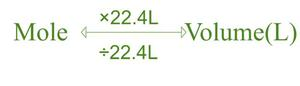# STP Formula

• Last Updated : 12 May, 2022

Standard temperature is defined as the freezing point of pure water at sea level, 0˚C (zero degrees Celsius), or 32˚F (32 degrees Fahrenheit) or 273˚K (273.15 degrees kelvin). Standard pressure is defined as a unit of pressure that will support a column of mercury 760 mm high at sea level and 0 degrees centigrade. The STP is defined as 0˚C and 1 atm. The volume of a gas depends on several factors. The gas’s temperature, the gas’s pressure, and the amount of gas (number of moles).

### STP Formula

The ideal gas law states that the volume occupied by a gas depends on the amount of substance (gas), temperature, and pressure. Standard temperature and pressure are 0 degrees Celsius and 1 atmosphere of pressure. Parameters of gases are significant for calculations in chemistry and physics and are usually calculated at STP.

By using the law of Ideal gas,

P × V = n × R × T

Where as, P = Pressure

V = Volume

n = no. of moles

R = molar gas constant

T = Temperature

At STP

T = Tstp = 273.15 K

P = Pstp = 1 atm and

n = 1 mol gas

R = 0.08206 L-atm/mol-K

V = Vstp = (n × R × Tstp)/Pstp

= ((1 mol) × (0.08206 L-atm/mol-K))/(1 atm)

= 22.414 L / mol

### Sample Questions

Question 1:  What is the volume of oxygen that, as 2.50 mol, exerts a pressure of 1500 mm mercury at 20 degrees C?

Solution:

We know that from the definition, 1∙atm will support a column of mercury that is 760∙mm high. We use Kosher units,

i.e., P = (1500 mm. Hg)/(760 mm. atm-1)

= 1.97 atm.

Then, we solve for volume in the Ideal Gas Equation,

V = (n × R × T)/P

= ((1.50∙mol) × (0.0821∙L∙atm/K∙mol) × (293∙K))/(1.97∙atm)

= 30.5 L.

Question 2: Is the volume of 25 g dinitrogen monoxide gas at STP?

Solution:

Nitrogen has a molar mass of 14.0067 g/mol, and oxygen has a molar mass of 15.999 g/mol.

Molar mass of N2O = ((2 × 14.0067) + 15.999) g/mol = 44.0124 g/mol.

The molar volume of gas at STP is 22.4 L/mol.

Thus, the volume of 20.5 g of N2O is,

= ((22.4 L/mol) × (25 g)) /(44.0124 g/mol)

= 12.7 L

Question 3: What is the volume of 5 moles of Carbon dioxide gas at STP?

Solution:

An ideal gas at STP occupies 22.4 L.

Assuming co2 behaves ideally 5 moles,

= 22.4 L × 5 = 112 L.

Question 4: The gram equivalent volume of O2 at STP?

Solution:

The gram equivalent mass of oxygen is 16.

32 g of Oxygen occupies 22.4 L at STP.

So, applying simple unitary method, the gram equivalent volume of oxygen will be 11.2 L.

Question 5: Is the volume of 55g of Ozone or trioxide at STP?

Solution:

The volume of one mole of a gas is 22.414 L/mol.

You need to calculate the number of moles in 55 g O3 using the formula n = m/M, where,

n = mole =?

m = mass = 55 g O3

M = molar mass = 3×15.999 g/mol O3 = 47.997 g/mol

n = (55 g O3/47.997 g/mol) = 1.145 mol O3

Calculate volume of O3 by multiplying mol O3 by the Vm.

= (1.145 mol O3×22.414 L/mol) = 25 L

The volume of 55 g of O3 at STP is 25 L.

Question 6: What is the volume of gas in STP in cm3?

Solution:

1 mol of gas has a volume = 22.4 L.

We know that there are 1000cm³ in 1L.

The volume of 1mol gas at STP

= 22.4L × 1000cm³/L

= 22,400cm³

Question 7: Where Argon gas occupies 50 L determines the number of moles and mass of the argon sample?

Solution:

One mole of gas = 22.4L.Therefore,

= 50 L.Ar/22.4L

= 2.23 mol.Ar

We know that mass of argon = 39.95

= 2.23 mol × (39.95/ 1 mol.Ar)

= 89 g.Ar.

Question 8: What volume will 1.25 moles of Helium gas occupy at STP?

Solution:

One mole of gas = 22.4 L.Therefore,

= 1.25 mol × 22.4 L/mol

= 28.4 L.

My Personal Notes arrow_drop_up
Recommended Articles
Page :